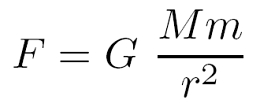# Why is there inertia

## inertia

Indolence is a quality of Masses. Inertia means that every body tries to remain in its state of motion and opposes a change in movement with resistance: Still objects oppose movement; moving objects offer resistance to deceleration or distraction. That is precisely the statement of the Law of inertia, the first of Newton's three laws. But why is it that masses are inert?

### Newtonian gravitational forceMasses are attracted by gravitational forces. The English physicist and mathematician could already do this Sir Isaac Newton in the 17th century with his Newton's law of gravitation occupy. The equation for the Gravitational force is on the right. Are in it G the gravitational constant with a numerical value of 6,672 × 10-11 m3 kg-1 s-2, M. and m two masses that are at a distance r like to be located. The force has the typical drop with it r-2 (like Coulomb's law of electrostatics). Physicists also speak of one 1 / r potential. The force is precisely the negative gradient of this potential.

### The long arm of gravity

This gravitational interaction has one infinite range. For large numerical values ​​of r the gravitational force becomes very small, but remains finite. Above all, the gravitational force can not only be shielded from charges of different polarity: there are no 'negative masses'! That is the main difference between gravity and electromagnetic force. The infinite range is also reflected in the fact that the (hypothetical) graviton of the quantum field theoriesRest mass zero have.
This long range has the consequence that ultimately all the crowds feel in the universe (theoretically). One can therefore say that the inertia of individual masses is a consequence of the fact that they interact with all other masses.

### Gravitational force takes time to spread

The interaction is, however not instantaneously, as Newton assumed, but propagates at finite speed, the Vacuum speed of lightc, out. Gravitational waves, as described in general relativity (GTR), propagate at the speed of light.

### Equivalence principle

The equivalence principle requires that inert and heavy mass equivalent be. So you cannot decide in a closed box whether it is at rest in a gravitational field or whether it is evenly accelerated by a constant force. The principle is one of the pillars of ART.

### Inertial forces

As inertial forces inertial forces) denotes forces that occur when a reference system is accelerated. Centrifugal force and Coriolis force are two inertial forces that occur in rotating frames of reference (which are not inertial). Inertial forces are also called Pseudo-forcesbecause their occurrence depends on the frame of reference. Inertial forces correct Newtonian or relativistic mechanics, as it were seen from different systems: One system is an inertial system and has an inertial observer in it. The other system rotates and is therefore a non-inertial system with a rotating, non-inertial observer. The rotating observer now feels inertial forces, for example when he is sitting in a carousel and the centrifugal force is pushing him outwards. But the inertial observer who looks at the carousel from outside, for example, does not feel any inertial forces. From his point of view, the inertial forces try to make the movement of the non-inertial observer inertial, i.e. straight.